# Implementation and Validation of a specific Bayesian Hierarchical Mixture of Experts Framework

Michael Anton Kraus

Michael Kraus did his honours project at the Specific Field Continuum Mechanics (TUM) (TUM) and
was supervised by Prof. Phaedon-Stelios Koutsourelakis, PhD.

#### Introduction and Background

The representation of data, generated by an unobserved process, is at the core of Pattern Recognition and Machine Learning. This project deals with combining the two Machine Learning tasks “Classification” and “Regression” via a Hierarchical Mixture of Experts in a Bayesian fashion. The machine learning approach to modelling data constructs models by beginning with a flexible model, specified by a set of parameters, and then finds the setting of these model parameters that explains or fits the data best. What is meant by ’best’ depends on the choice of the user and is in the probabilistic context mainly associated with finding a Maximum Likelihood (ML), Maximum A Posteriori (MAP) or minimum Kullback-Leibler-Divergence (in the Variational Bayesian framework, ) estimate of the parameter setting, c.f. , .

#### Bayesian Hierarchical Mixture of Experts (HME)

The architecture of a HME is a tree with a gating network at the nonterminals of the tree and expert nodes which sit at the leaves of the tree. Each expert produces an output for each input x . The output is processed up the tree being blended by the scalar gating network outputs, which depend also on the input x . The data are assumed to form a countable set of paired observations X = (x(n), y(n)), . The basic element of the tree is a gating node with two associated expert nodes, this is ilustrated with a box in Figure 1. In the context of this project, the formulas for learning the parameters of the Experts, which will be Probabilistic Principal Component Analysers (PPCA) regressors, and of the gating network, which will consist of Probit classifyers, were derived and implemented. We further enhanced the standard HME to a Generalized HME as we used variational techniques for finding approximate posterior distributions on the HME parameters. The reason for using a variation approach was, that the author did not want to retrain the whole tree everytime it expands with two new leaves.

#### Implementation

The Parts of the object-oriented implementation of the HME framework as well as the overall workflow in terms of called functions is illustrated in the following figures: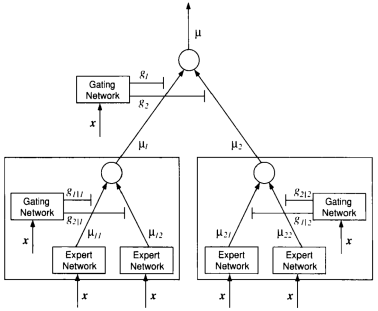Fig.1: Hierarchical Mixture of Experts, c.f. 4

In order to keep this outline short, not all details of each function, which was shown in the above figure, will be explained here. Instead we will here describe the general workflow with the implemented framework: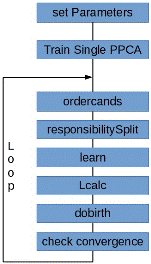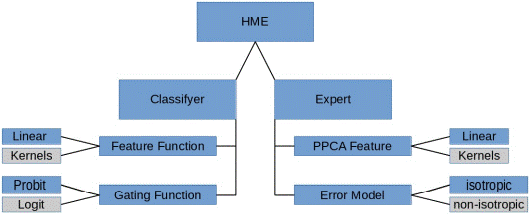(a) Parts of the Generalized HME Framework (b) Level-set

Fig.2: General Workflow with the implemented Generalized HME Framework

In a first step, the user has to preset some overall training parameters (e.g. values for the parameter priors, etc.), then one single PPCA is trained in order to be able to suggest a first responsibility split. The function ’ordercands’ performs a selection of a node out of the whole set of candidates (leaves of the current tree) for the split. When the node to split is selected, the function ’responsibilitySplit’ takes over and performes a split of the parent posterior responsibility and assigns the responsibilities to the two children. The function ’learn’ is the core of the HME framework as it trains the parameters of the Classifyer and the Experts. The function therefore naturally has two components, a function for training the Classifyer and a function for training the Experts. As we want to use the Expectation-Maximization-Algorithm to find the parameter values, each training function calls alternatingly an E-Step function and then a M-Step function. The learning is finished as soon as some convergence criteria are met. The learning process is followed by the calculation of the Loglikelihood via the function ’Lcalc’. Having at hand the Loglikelihood and the parameters of the HME, we can decide whether to accept or reject the proposed split via the function ’dobirth’. Finally the exists a function ’check convergence’ which monitors the overall convergence of the tree in terms of the algorithm explored the space of all allowed model trees.

#### Numerical Examples

The implemented HME framework was validated against 5 Test-Cases of increasing complexity in terms of the nonlinearity of the classification boundary. Here only the setup and results for Test Case 4 are presented. The data were sampeled from a model tree, which consists of one nonlinear Classifyer Gating Node and two PPCA Experts. The configuration is shown in the following picture: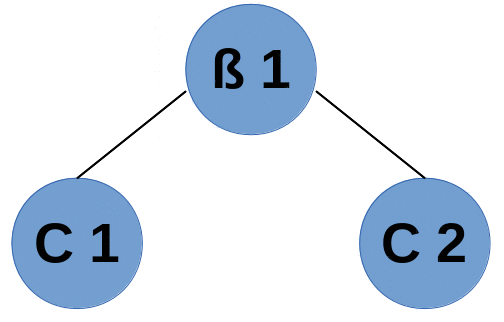Fig.4: Model Tree

In the data (y) space, the two PPCAs are Gaussians, which are illustrated below: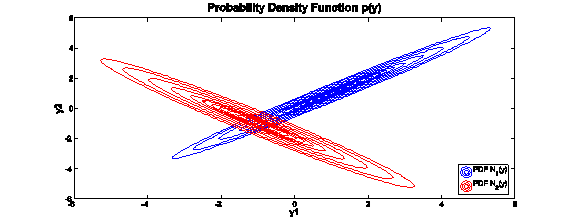Fig.4:Probability Distribution FunctionsofthetwoGaussians in dataspace

In the input (x) space, the Classification Boundary is given by an circle, which can be seen below: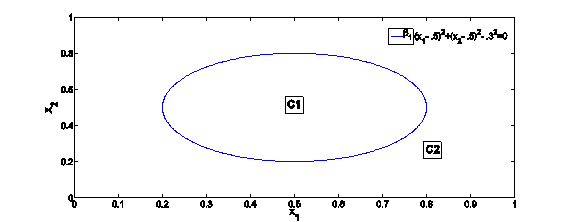Fig.4:Partitioning in x (input) space

The results from the Expectation – Maximization – training of a linear classifyer and two PPCA experts in terms of accuracy of the found parameters against the ground truth and in terms of the predictive Likelihood are shown in the following figures: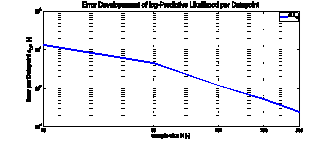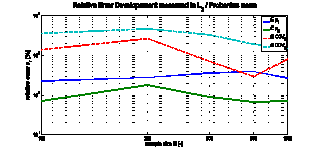(a) Error per Datapoint LDP over the training sample size N (b) Relative Error in the Model Parameters

#### Conclusions

The training was redone with modifyed linear classifyers and kernels, but the results are not shown here. Further details about the implementation and the other conducted Test Cases can be found in .

#### References

  Christopher M. Bishop Pattern Recognition and Machine Learning Springer, 2006.  M. J. Beal Variational Algorithms for Approximate Bayesian Inference. PhD thesis, 2003.  Figueiredo, M.A.T. Adaptive sparseness for supervised learning IEEE Transactions on Pattern Analysis and Machine Intelligence, 25, no.9, 1150- 1159, Sept. 2003.  Michael I. Jordan, Robert A. Jacobs. Hierarchical Mixtures of Experts and the EM Algorithm Neural Computation, 6,181-214,1994.  Michael A. Kraus. Implementation and Validation of a specific Bayesian Hierarchical Mixture of Experts Framework Honours-Project for BGCE, March 2014.